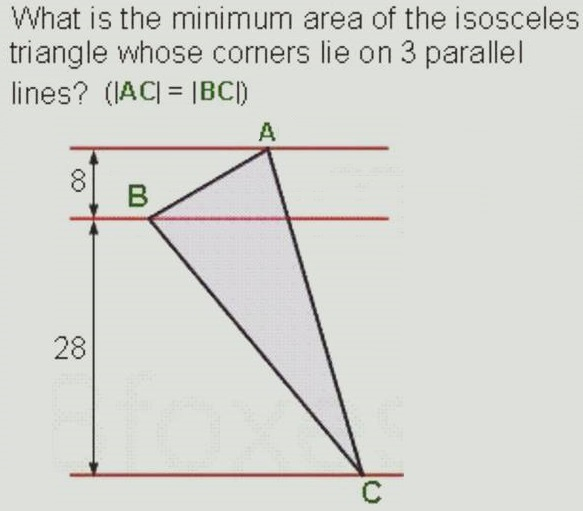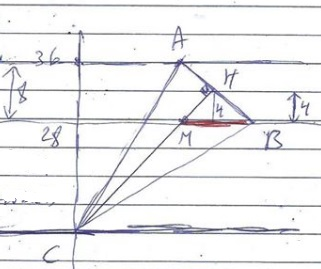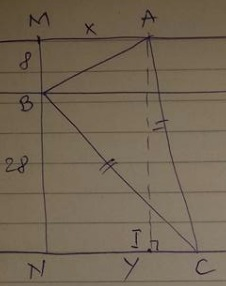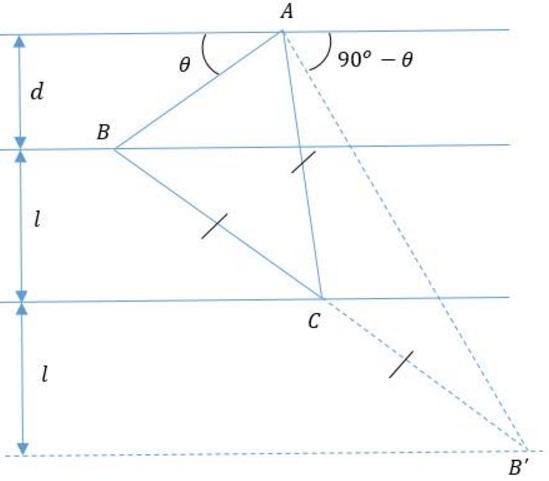# Area of Isosceles Triangle

### Source### Solution 1

Assume $A=(a,36),\;$ $B=(b,28),\;$ $C=(0,0).$Then, by the Pythagorean theorem, $BC^2=b^2+28^2,\;$ $AC^2=a^2+36^2.\;$ Since the triangles is isosceles, $a^236^2=b^2+28^2,\;$ or $b^2=a^2+512.$

We now compute the cross-product of two vectors $\overrightarrow{CA}\times\overrightarrow{CB}\;$ whose norm gives twice the area of $\Delta ABC.$

$\displaystyle \overrightarrow{N}=\overrightarrow{CB}\times\overrightarrow{CA}=\left|\begin{array}\;\overrightarrow{i}&\overrightarrow{j}&\overrightarrow{k}\\ b&28&0\\a&36&0\end{array}\right|=(36b-28a)\overrightarrow{k}=4(9b-7a)\overrightarrow{k}.$

Let $\displaystyle f(a)=\frac{||\overrightarrow{N}||^2}{16}=130a^2+41472-126a\sqrt{a^2+512}.\;$ $\min f(a)=16384=128^2=f(28).$ It follows that the minimum of $\displaystyle [\Delta ABC]=\frac{1}{2}\cdot 4\cdot 128=256.$

### Solution 2

In right $\Delta BHM,\;$ $\displaystyle 4\cdot BM=HM\cdot BH\le\frac{1}{2}(HM^2+BH^2)=\frac{1}{2}BM^2,\;$ implying $BM^2\ge 8\cdot BM,\;$ i.e., $BM\ge 8.$Further,

\displaystyle \begin{align} [\Delta ABC]&=2[\Delta CHB]\\ &=2([\Delta CMB]+[\Delta BHM]\\ &=2\left(\frac{1}{2}BM\cdot 28+\frac{1}{2}BM\cdot 4\right)\\ &=2\cdot 16\cdot BM. \end{align}

It follows that $[\Delta ABC]= 32\cdot BM\ge 32\cdot 8=256.\;$ Observe that at the minimum $HM=HB,\;$ making $\Delta BHM\;$ right isosceles.

### Solution 3$BC^2=28^2+y^2,\;$ $AC^2=36^2+(y-x)^2,\;$ so that $36^2+(y-x)^2=28^2+y^2,\;$ from which $\displaystyle y=\frac{512+x^2}{2x}.$

$\displaystyle S=\frac{(x+y)\cdot 36}{2}-4x-14y.\;$ In other words, $\displaystyle S(x)=\frac{16x^2+1024}{x}.\;$ $S'(x)=0\;$ at $x=\pm 8.\;$ It is positive only for $x=8:\;$ $S(8)=256.$

### Solution 4\begin{align} [\Delta ABC]&=[PQCR]-[\Delta AQC]-[\Delta BRC]-[\Delta ABP]\\ &=36(x+y+z)-18(y+z)-14(x+y+z)-4x\\ &=18x+4y+4z. \end{align}

On the other hand, $[\Delta ABC]=[\Delta ABS]+[\Delta BCS]=18x+18y.\;$ Comparing the two gives $4y+4z=18y,\;$ or $\displaystyle z=\frac{7y}{2}.$

Now, since $AC=BC,\;$ $28^2+(x+y+z)^2=36^2+(y+z)^2\;$ which translates to

\begin{align}512&=x(x+2y++2z)\\ &=x(x+2y+7y). \end{align}

Thus, $\displaystyle 9y=\frac{512}{x}-x.\;$ It follows that

\displaystyle\begin{align} [\Delta ABC]&=18x+18y=18x+\frac{1024}{x}-2x\\ &=16x+\frac{1024}{x}\\ &\ge 2\sqrt{2^4\cdot 2^{10}}\\ &=2^8=256. \end{align}

### Solution 5The equation of the perpendicular bisector of $AB\;$ cab ne found to be $\displaystyle y-4=\frac{-b}{8}\cdot\frac{c-b}{2},\;$ from which $\displaystyle c=\frac{b^2+32\cdot 16}{2b}.\;$ Further,

$\displaystyle A=[\Delta ABC] =\frac{1}{2}\left|\begin{array}{ccc}\;0&0&1\\ b&8&1\\c&-28&1\end{array}\right|=4c+14b=\frac{2(b^2+3\cdot 16)}{b}+14b=16b+\frac{32^2}{b}.$

$A'=0\;$ for $b=8\;$ such that $A''(8)\gt 0,\;$ implying minimum at $b=8.\;$ For $b=8,\;$ $c=36\;$ such that $A=4\cdot 36+14\cdot 8=256.$

### Solution 6$\displaystyle CM=\frac{32}{\sin\alpha},\;$ $\displaystyle AB=\frac{8}{\cos\alpha}.\;$ Hence,

$\displaystyle [\Delta ABC]=\frac{1}{2}AB\cdot CM=\frac{256}{\sin 2\alpha}\ge 256.$

The minimum is achieved with $\sin 2\alpha=1,\;$ i.e., for $\alpha=45^{\circ},$ remarkably independent of the rest of the data!

### Solution 7### Solution 8Set $r = AC = BC,\;$ $M\;$ the midpoint of $AB,\;$ $\varphi\;$ the angle between $CM\;$ and left horizontal, $\psi=\angle ACM.\;$ The equations area = $r^2\cos\psi\sin\psi,\;$ $r\sin(\varphi-\psi) = 28,\;$ $r\sin(\varphi+\psi) = 36\;$ lead easily to area being $256\csc(2 \varphi)\;$ with minimum $256\;$ at $\varphi =\displaystyle\frac{\pi}{4}.$

### Solution 9By the construction, $CA=CB=CB',\;$ implying $\angle BAB'=90^{\circ}.\;$ It follows that

\displaystyle\begin{align} [\Delta CAB]&=\frac{1}{2}[\Delta BAB']\\ &=\frac{1}{4}AB\cdot AB'\\ &=\frac{1}{4}\frac{d}{\sin\theta}\cdot\frac{d+2l}{\sin(90^{\circ}-\theta )}\\ &=\frac{d(d+2l)}{4\sin\theta\cos\theta}\\ &=\frac{d(d+2l)}{2\sin 2\theta}\\ &\ge \frac{d(d+2l)}{2}. \end{align}

The minimum is achieved for $\theta = 45^{\circ},\;$ at which time $\displaystyle[\Delta ABC]=\frac{1}{2}d(d+2l)=256,\;$ for $d=8\;$ and $l=28.$

### Solution 10By the construction, $CA=CB=CB',\;$ implying $\angle BAB'=90^{\circ}.\;$ Let $M\;$ be the midpoint of $BD.\;$ It follows that

\displaystyle\begin{align} [\Delta CAB]&=\frac{1}{2}[\Delta BAB']\\ &=\frac{1}{4}(d\cdot BD+2l\cdot BD)\\ &=\frac{1}{4}((d+2l)\cdot BD)\\ &=\frac{1}{4}((d+2l)\cdot 2\cdot AM)\\ &=\frac{1}{2}((d+2l)\cdot AM)\\ &\ge \frac{d(d+2l)}{2}. \end{align}

The minimum is achieved when $AM\perp CD,\;$ at which time $\displaystyle[\Delta ABC]=\frac{1}{2}d(d+2l)=256,\;$ for $d=8\;$ and $l=28.$ Condition $AM\perp CD\;$ is equivalent to $\Delta ABD\;$ being isosceles.

### Acknowledgment

The problem has been posted to the CutTheKnotMath facebook page by Imad Zak, along with two solutions (Solution 1 and Solution 2). Imad Zak has generously shared the problem previously posted at his Math facebook group by María Jesús Méndez. From his group I have borrowed additional solutions: Solution 3 by Xhevahir Tafciu, Solution 4 by Soumava Chakraborty, Soution 5 by María Jesús Méndez; Solution 6 is by Marco Antônio Manetta, Solution 7 by Kunihiko Chikaya; Solution 8 is by Grégoire Nicollier; Solutions 9 and 10 are by Emmanouil Lamprakis.

Note that the three trigonometric solutions (6, 8 and 9) show that the result is independent of the numeric data. Solution 10 also leads to this observation.

### A Sample of Optimization Problems

• Mathematicians Like to Optimize
• Reshuffling knights - castle defenders
• Isoperimetric Theorem and Inequality
• Viewing a Statue: the Problem of Regiomontanus
• Fagnano's Problem
• Minimax Principle Demonstration
• Maximum Perimeter Property of the Incircle
• Extremal Problem in a Circular Segment
• Optimization in Four Variables with Two Constraints
• Daniel Dan's Optimization in Three Variables
• Problem in a Special Trapezoid
• Cubic Optimization with Linear Constraints
• Cubic Optimization with Partly Linear Constraints
• Problem M317 from Crux Mathematicorum
• Area of Isosceles Triangle
• Minimum of Cotangents from Saint Petersburg
•